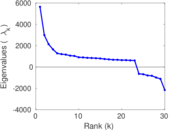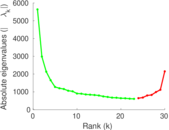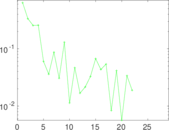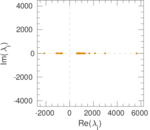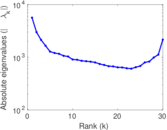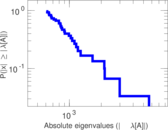This asymmetric network contains Twitter follow data based on a snapshot taken in 2009. A node represents a user. A directed edge indicates that a user follows another user.

 Code `TF` Internal name `twitter_mpi` Name Twitter (MPI) Data source http://twitter.mpi-sws.org/ AvailabilityDataset is available for download Consistency check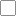Check was not executed Category Online social network Dataset timestamp 2010 Node meaning User Edge meaning Following Network format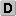Unipartite, directed Edge typeUnweighted, no multiple edges ReciprocalContains reciprocal edges Directed cyclesContains directed cycles LoopsDoes not contain loops Snapshot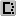Is a snapshot and likely to not contain all data

## Statistics

 Size n = 52,579,682 Volume m = 1,963,263,821 Loop count l = 313 Wedge count s = 177,464,933,477,000 Triangle count t = 55,428,217,664 Maximum degree dmax = 3,691,240 Maximum outdegree d+max = 779,958 Maximum indegree d−max = 3,503,656 Average degree d = 74.677 7 Size of LCC N = 52,515,193 Diameter δ = 18 50-Percentile effective diameter δ0.5 = 3.151 81 90-Percentile effective diameter δ0.9 = 4.052 51 Mean distance δm = 3.615 64 Clustering coefficient c = 0.000 937 000 Operator 2-norm ν = 6,971.10 Reciprocity y = 0.355 691 Non-bipartivity bA = 0.380 728

## Plots

### Degree distribution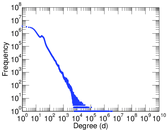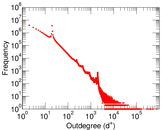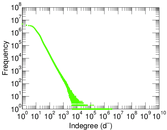### Cumulative degree distribution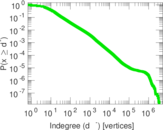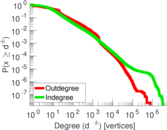### Spectral graph drawing based on the adjacency matrix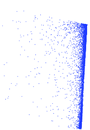### Hop distribution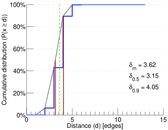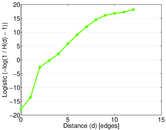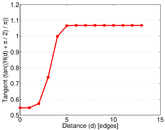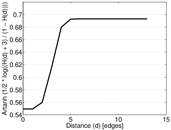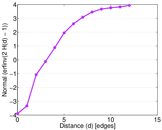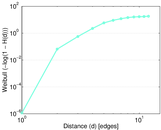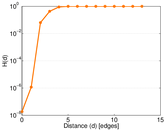### Matrix decompositions plots